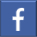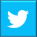# Random Module-Python

## This blog gives in-depth knowledge of Python Random Module methods. Read our blog to learn more...Oct 08, 2022    By Team YoungWonks *

## What is Python Random Module?

A random action is one that is carried out without regard for any particular method. Python's random module is built-in. It is used to code by performing actions at random. The probability of selecting anything from the given range remains constant. For example, pseudo-random numbers such as random integers, random decimal values, or selecting a random item from a list can be used.

In this Python tutorial, we'll go over all of the functions available in the Python random module and how to use them in code.

## Basic Requirement

Import the module before using the functions.

Syntax: import random

## Python Random Module Functions

### 1. Random Integers

a. This first random number generator picks integers randomly from a given range.

Syntax: random.randint(start, end)b. The second method is used to pick a random number from a specified range having the step value as well.

Syntax: random.randrange (start, stop, step)### 2. Random decimal numbers(float)

a. This methods picks random floating point number from a given range.

Syntax: random.uniform(start, end)b. Random decimal numbers from 0 to 1: This method picks random float number between 0 to 1.

Syntax: random.random()### 3. Random Element Picker

Apart from the above pseudo-random number generators, we can use the random module to pick random element from a list, tuple or string.

Syntax: random.choice(list)The above method picks a random item from the list.

### 4. Random Element Picker as a list

In order to generate a list of random items we can use the method given below.

Syntax: random.sample(n, list)

Here, n is the required number of items### 5. Random Jumble the list

We can randomly jumble the items of a list using the random module.

Syntax: random.shuffle(list)### 6. Random Seed

The seed method is used to initialize the random number generator. It also allows us to generate the same random number where the start seed value is always the current system time.

Syntax: random.seed()### 7. Random State

In Python, the Random module includes functions for setting and retrieving the state. It obtains or sets the current thread's state. Threading is the simultaneous execution of multiple smaller operations.

Syntax: random.getstate()

random.setstate(value)### 8. Random Bits

We can find the size of the randomly picked integer or element.

Syntax: random.getrandbits(value)### 9. Random Triangular Values

This method returns a random float number from the specified range using a triangular random function. The third parameter is the mode, which is the midpoint of the numbers specified. It does not provide bias to either extreme value by default. However, if the mode value is included, the generated random number will be biased towards it.

Syntax: random.triangular (start, step, mode)

### 10. Types of Distribution random values

There are a few other random module functions that are used for statistics. It is used to generate random values based on the type of distribution's. To visualize the distribution, we can use the random float numbers generated to generate a graph with the matplotlib module. This is commonly used in data science algorithms.

Let us now look into these inbuilt methods and their syntax: These methods generate random float numbers between 0 to 1.

a. Beta Distribution: This is a probability distribution of value from a finite range.

Syntax: random.betavariate()Here, we can also observe the beta distribution graphically using matplotlib to understand its applications.b. Exponential Distribution: This is the probability distribution of the amount of time until specific task is happens.

Syntax: random.expovariate()

Mean of the exponential distribution is 1/lambdac. Gaussian Distribution: This is also known as the normal distribution. This is a bell-shaped curve where equal number of values lie below and above the mean of all the values.

Syntax: random.gauss()

random.normalvariate()d. Logarithm Distribution: In this method, the natural log of the random values are normally distributed.

Syntax: random.lognormvariate()

e. Gamma Distribution: This method is used to analyze the continuous variables which also has skewed distributions. This is majorly used in data science.

Syntax: random.gammavariate()f. Von Mises Distribution: This is also known as the Fisher-von Mises distribution. It is used to specify the maximum entropy distribution of the circular movement.

Syntax: random.vonmisesvariate()

g. Pareto Distribution: random.paretovariate()

h. Weibull Distribution: In statistics, this probability distribution is used to observe the failure of a phenomenon. It analyses product reliability, life data, and model failure.

Syntax: random.weibullvariate()

## Summary

Python's random module is one of the most versatile modules in the language. It is used to derive and analyze results in many fields such as data science, statistics, probability, and mathematics. Now that you've learned these methods, you can use them in your code.*Contributors: Written by Aayushi Jayaswal; Lead image by Shivendra Singh

This blog is presented to you by YoungWonks. The leading coding program for kids and teens.

YoungWonks offers instructor led one-on-one online classes and in-person classes with 4:1 student teacher ratio.

Sign up for a free trial class by filling out the form below:

By clicking the "Submit" button above, you agree to the privacy policyShare on FacebookShare on Twitter
help